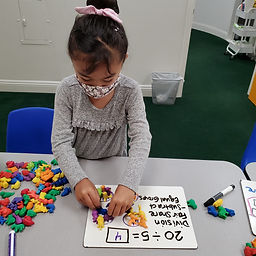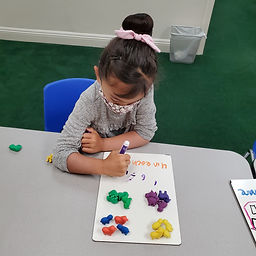## Ms. Raejean

### Target 1​

###### Lesson Type:

New

Number Operation

:

Computation

Divide within 100.

###### 1:

Understand that dividing numbers makes numbers smaller.

###### 2:

Understand that division represents taking a quantity and determining how many equal groups of objects can be made.

###### 3:

Understand there are multiple ways to divide a multi-digit number.

3rd

###### Vocabulary:

Division, "Fair Share," "Equal Groups"

Activities:

Division was introduced. Students used animal counters to "fair share," as well as make "equal groups." Students practiced writing the equation and division symbol. Students also explored different ways to divide the same group. (Ex. 12 can be broken up into 2 groups of 6 or 3 groups of 4)### Home Exploration

###### Guiding Questions:## Absent Students:

### Target 2

:

###### 1:

Compose larger shapes from smaller shapes.

###### 2:

Using triangles in a tangram set, make triangles, squares, and parallelograms.

###### 3:

Using all seven pieces in a tangram set, make squares, parallelograms, triangles, trapezoids, and rectangles.

3rd

###### Vocabulary:

Tangrams, Composite/Compose

Activities:

Students used tangrams to make larger shapes. 2 triangles were used to make a square, parallelogram, and triangle. 7 tangrams were used to try to make a large trapezoid.### Home Exploration

###### Guiding Questions:### Target 3

:

###### Vocabulary:

Activities:### Home Exploration